# Seeing the World in Code: First Glimpse at Image Processing

255. It’s a number I’ve seen for the longest time and only ever considered it as a value that I enter in dialog boxes on some photo-editing software. However, using Python code to perform image processing has definitely given a new meaning to “255” along with everything I know and do not know about digital images.

et us begin by Zooming into a 2x2 black and white image. In order for us to visualize this, we will use numpy as we will mostly process images using their numeric array form.

`import numpy as npfrom skimage.io import imshow, imread`

For a black and white image, 255 means white and 0 means black. Thus, with the following array:

`array = np.array([[255, 0],                   [0, 255]])`

We then view this as an image:

`imshow(array, cmap='gray');`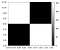Figure 1. 2 by 2 grayscale image showing black and white

And in order for us to show grays, we simply have to use any value in between 0 and 255.

`grays = np.random.randint(0, 256, (3,5))grays`
`imshow(grays, cmap='gray');`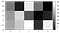Figure 2. 3 by 5 grayscale image showing random levels of gray based on an array with values between 0 to 255

We can also achieve this by using an array of floating point numbers with values from 0 to 1.

`grays_2 = np.random.random((3,5))grays_2`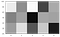Figure 3. 3 by 5 grayscale image showing random levels of gray based on an array of floating point numbers

## “In nature, light creates the color. In the picture, color creates the light.” — Hans Hofmann

After looking at black, whites, and grays, let’s now add some color. Colored digital images are basically just made up of the red, green, and blue or RGB channels. Thus, from a 2-dimensional array, we add another dimension or a depth of 3 — one for each channel. Implementing that idea in code:

`rgb = np.random.randint(0, 256, (3,3,3))rgb`

This is then interpreted as a 3 by 3 image with 3 color channels producing the following image:

`imshow(rgb);`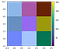Figure 4. 3 by 3 RGB image showing random colors based on a 3 by 3 by 3 array of values between 0 to 255

Something that will come in handy in our future image processing is separating images into their individual channels. In order to do that, we just index the array on the third dimension.

`red = rgb[:,:,0]green = rgb[:,:,1]blue = rgb[:,:,2]`

We can then visualize these channels individually. So, with our 3 by 3 RGB image above, we have the following:

`import matplotlib.pyplot as pltfig, ax = plt.subplots(1, 3, figsize=(12,3))ax.imshow(red, cmap="Reds")ax.set_title('Red Channel')ax.imshow(green, cmap="Greens")ax.set_title('Green Channel')ax.imshow(blue, cmap="Blues")ax.set_title('Blue Channel');`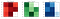Figure 5. Image from Figure 4 separated into its R, G, and B channels

We can observe, for example, that where there are lighter values of one channel, another dominates such as both corners on the right with a bluish green on the lower right, and red on the upper right. We can also see that where the colors are darkest, when displayed as one image, it is closest to white.

# Looking at Things in a Different Light

Apart from the RGB colors space we can also represent images using a different color space. This is the HSV color space. H stands for hue which to put it simply, contains all the colors of the rainbow. S stands for saturation which is basically how strong (or dull) the colors are. Finally, V stands for value which is the brightness or darkness the colors or the image. To demonstrate this let’s use the following image:

When using `imread`, the values of the arrays default to RGB values. While we’re here, let’s visualize the separated RGB channels for comparison later:

`sunrise = imread('sunrise.jpg')fig, ax = plt.subplots(1, 3, figsize=(12,4))ax.imshow(sunrise[:,:,0], cmap='Reds')ax.set_title('Red')ax.imshow(sunrise[:,:,1], cmap='Greens')ax.set_title('Green')ax.imshow(sunrise[:,:,2], cmap='Blues')ax.set_title('Blue');`

We then convert the RGB image into HSV, or more accurately, we derive the . Fortunately, there is already a `scikit-image` package for this purpose.

`from skimage.color import rgb2hsvsunrise_hsv = rgb2hsv(sunrise)`

We then visualize the HSV channels individually similar to how we would plot the individual RGB channels.

`fig, ax = plt.subplots(1, 3, figsize=(12,4))ax.imshow(sunrise_hsv[:,:,0], cmap='hsv')ax.set_title('Hue')ax.imshow(sunrise_hsv[:,:,1], cmap='gray')ax.set_title('Saturation')ax.imshow(sunrise_hsv[:,:,2], cmap='gray')ax.set_title('Value');`

As we can see the picture actually only has a few hues. However, both saturation and value channels show a great deal of variety. Thus, we can conclude that the texture of the clouds, for example, can be derived from having various saturation and brightness of the hues between red and yellow.

Hopefully, this gave you a better idea on the numbers and code behind each image so when you do Image Processing, you’ll have some direction on what you want to achieve.

Please visit my blog post on Image Enhancements where we apply what we’ve learned so far and augment these with new techniques to enhance images through white balancing and histogram manipulation and.

Am I doing this data science thing right?

## More from Tonee Bayhon

Am I doing this data science thing right?